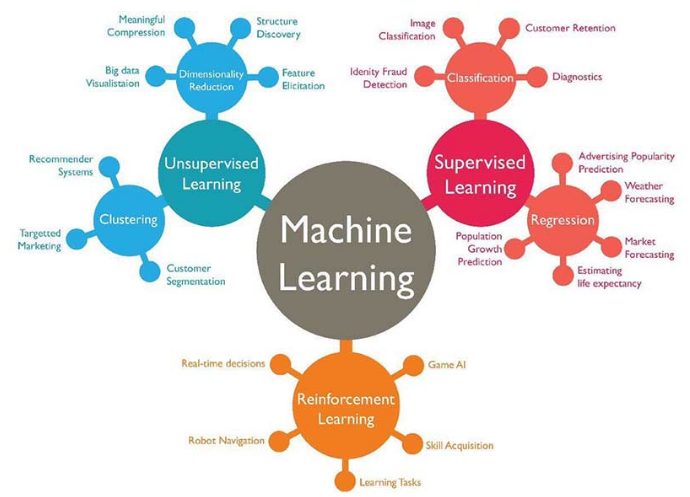# Navigating the World of Machine Learning: A Comprehensive Guide to Learning and Mastering ML Algorithms### Introduction

Mastering Machine Learning (ML) algorithms is a cornerstone for anyone aiming to thrive in the field of data science and artificial intelligence (AI). These algorithms provide the engine that drives AI systems, enabling them to learn from data, make predictions, and improve over time. This guide takes a detailed look at the steps involved in learning a machine learning algorithm, offering valuable insights and tips to aid your journey.

### Understanding Machine Learning Algorithms

At its core, a machine learning algorithm is a procedure that is used to process data and make predictions or decisions without being explicitly programmed to do so. These algorithms enable machines to learn from data, draw conclusions, and make predictions or decisions. There are numerous types of machine learning algorithms, each with its unique characteristics, advantages, and disadvantages.

### How to Learn a Machine Learning Algorithm

Learning a machine learning algorithm requires a systematic approach. Here are some steps to consider:

Understand the Intuition: Begin with grasping the fundamental intuition behind the algorithm. Understand what problem it solves, how it works, and why it works. Understanding these core concepts can help you apply the algorithm more effectively.

Learn the Mathematics: Machine learning algorithms are deeply rooted in mathematics. Take time to understand the mathematical concepts and principles that underpin the algorithm. These could include areas such as linear algebra, calculus, statistics, and probability theory.

Process and Assumptions: Each algorithm makes specific assumptions about the data. Understand these assumptions and the process of the algorithm. This will help you in data preparation and understanding the suitability of the algorithm for a particular problem.

Implementation: Get hands-on experience by implementing the algorithm. Use programming languages like Python or R and ML libraries like scikit-learn, TensorFlow, or PyTorch to practice building models using the algorithm.

Experiment with Datasets: Once you’re comfortable with implementing the algorithm, experiment with different datasets. Try to use datasets from different domains to understand how the algorithm performs under various scenarios.

Evaluation: Understand how to evaluate the performance of the algorithm. Familiarize yourself with different evaluation metrics and learn how to interpret them. This step is crucial in determining the effectiveness of your model.

Tune the Algorithm: Machine learning algorithms come with hyperparameters that control the learning process. Learn how to tune these hyperparameters to optimize the algorithm’s performance.

### Common Types of Machine Learning Algorithms

There are many types of machine learning algorithms, but the most commonly used ones can be grouped into three categories:

Supervised Learning Algorithms: These algorithms learn from labeled training data and are used for tasks like regression and classification. Examples include Linear Regression, Decision Trees, and Support Vector Machines.

Unsupervised Learning Algorithms: These algorithms find hidden patterns or intrinsic structures in data. They are used for tasks like clustering and dimensionality reduction. Examples include K-Means Clustering and Principal Component Analysis.

Reinforcement Learning Algorithms: These algorithms learn to make decisions by interacting with an environment where certain actions are rewarded. They are used in fields like robotics and game theory.

### Conclusion

Learning machine learning algorithms is a rewarding journey that opens up numerous possibilities in the field of data science and AI. Understanding these algorithms, their mathematical underpinnings, and their practical implementation will empower you to build robust and effective machine learning models. This comprehensive guide provides a roadmap to learning machine learning algorithms, equipping you with the knowledge and skills to excel in this fascinating field.

# Personal Career & Learning Guide for Data Analyst, Data Engineer and Data Scientist

## Applied Machine Learning & Data Science Projects and Coding Recipes for Beginners

A list of FREE programming examples together with eTutorials & eBooks @ SETScholars

# Projects and Coding Recipes, eTutorials and eBooks: The best All-in-One resources for Data Analyst, Data Scientist, Machine Learning Engineer and Software Developer

Topics included:Classification, Clustering, Regression, Forecasting, Algorithms, Data Structures, Data Analytics & Data Science, Deep Learning, Machine Learning, Programming Languages and Software Tools & Packages.
(Discount is valid for limited time only)## Find more … …

Python Example – Write a Python program to find numbers between 100 and 400 (both included) where each digit of a number is an even number

Python Example – Write a Python function to create and print a list where the values are square of numbers between 1 and 30 (both included)

Excel formula for Beginners – How to Filter by date in Excel